# Geometry - math word problems

#### Number of problems found: 323

• Medians in triangle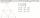Median of isosceles triangle has a length 3 cm. Determine the length of its sides if its perimeter is 16 cm.
• ParabolaFind the equation of a parabola that contains the points at A[6; -5], B[14; 9], C[23; 6]. (use y = ax2+bx+c)
• VectorCalculate length of the vector v⃗ = (9.75, 6.75, -6.5, -3.75, 2).
• CircleCircle is given by centre on S[-7; 10] and maximum chord 13 long. How many intersect points have circle with the coordinate axes?
• Circumscribing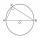Determine the radius of the circumscribed circle to the right triangle with legs 9 cm and 6 cm.
• CircleWrite the equation of a circle that passes through the point [0,6] and touch the X-axis point [5,0]: ?
• LineWrite an equation of a line parallel to To 9x + 3y = 8 That Passes Through The Point (-1, -4). Write in form ax+by=c.
• LineLine p passing through A[-10, 6] and has direction vector v=(3, 2). Is point B[7, 30] on the line p?
• SegmentsLine segments 62 cm and 2.2 dm long we divide into equal parts which lengths in centimeters is expressed integer. How many ways can we divide?
• Square side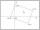Calculate length of side square ABCD with vertex A[0, 0] if diagonal BD lies on line p: -4x -5 =0.
• CircleFrom the equation of a circle: ? Calculate the coordinates of the center of the circle S[x0, y0] and radius of the circle r.
• CosineCalculate the cosine of the smallest internal angle in a right-angled triangle with cathetus 3 and 8 and with the hypotenuse 8.544.
• Vectors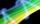For vector w is true: w = 2u-5v. Determine coordinates of vector w if u=(3, -1), v=(12, -10)
• CirclesHow many different circles is determined by 9 points at the plane, if 6 of them lie in a straight line?
• ClimbRoad has climbing 1:27. How big is a angle corresponds to this climbing?
• Perpendicular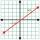What is the slope of the perpendicular bisector of line segment AB if A[9,9] and B[9,-2]?
• XY triangleDetermine area of triangle given by line 7x+8y-69=0 and coordinate axes x and y.
• Cone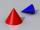If the segment of the line y = -3x +4 that lies in quadrant I is rotated about the y-axis, a cone is formed. What is the volume of the cone?
• Plane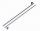On how many parts divide plane 6 parallels?
• SimilarityAre two right triangles similar to each other if the first one has a acute angle 70° and second one has acute angle 20°?

Do you have an interesting mathematical word problem that you can't solve it? Submit a math problem, and we can try to solve it.

We will send a solution to your e-mail address. Solved examples are also published here. Please enter the e-mail correctly and check whether you don't have a full mailbox.

Please do not submit problems from current active competitions such as Mathematical Olympiad, correspondence seminars etc...